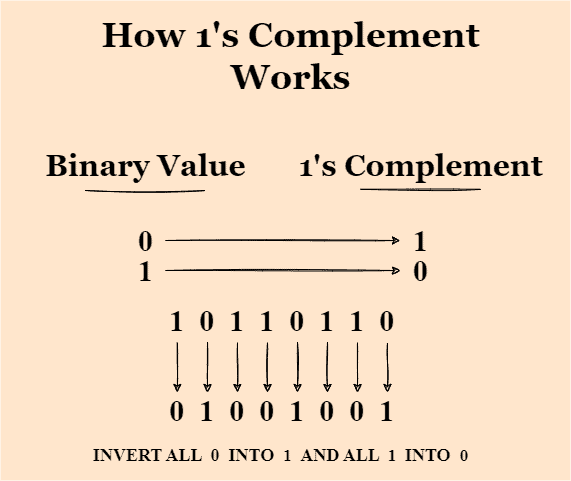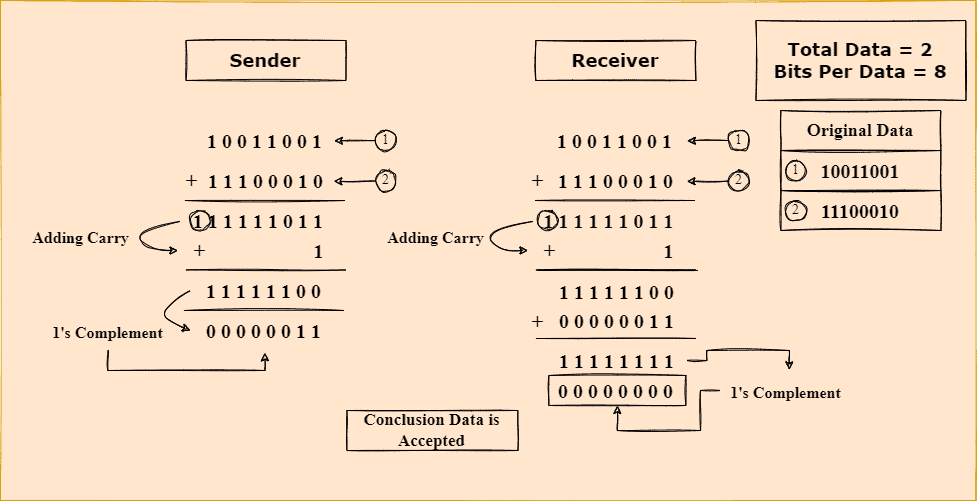## 1. Overview

In this tutorial, we’ll discuss how we can use 1’s complement technique to detect errors in data transmission.

Additionally, we’ll present a practical example of error detection using 1’s complement technique.

## 2. Introduction to 1’s Complement

Error detection is a critical part of any digital system that needs to maintain data integrity. Therefore, it’s essential to have a reliable method for detecting errors in transmitted data before it reaches its destination. One of the most commonly used techniques is 1’s complement. It’s a fast and efficient way of detecting errors in digital data.

In the 1’s complement system, each digit in a binary number is inverted. Hence, to find the 1’s complement of a binary number, we can simply replace each 1 with a 0 and each 0 with a 1. For example, to find the 1’s complement of 10110110, we replace the 1’s with 0’s and the 0’s with 1’s to get 01001001:We can also use 1’s complement to represent negative numbers in computers since it allows for simple addition and subtraction of binary numbers using standard circuits. Additionally, we can also utilize this technique to simplify the implementation of certain computer operations, such as bitwise NOT and bit shifting.

The 1’s complement of a binary number can be used for error detection when transmitting data and reversing the order of data to calculate a checksum. We can use this technique when converting a decimal number to a binary and vice versa. It can identify the most significant and least significant digits of a decimal number. If we want to find 1’s complement of a decimal number, we need to add all the digits together and then add a 1 to the result.

## 3. Error Detection Using 1’s Complement

Before discussing how we use 1’s complement technique for error detection, let’s discuss the different types of errors that can occur while transferring data.

There’re several different types of errors that can occur in digital data. Some common errors include single-bit, burst, timing, and corruption.

Single-bit errors affect a single bit in a digital data stream. Various factors, including interference, noise, or hardware malfunction, can cause these errors. Burst errors affect multiple consecutive bits in a digital data stream. Furthermore, timing errors occur when the timing of digital data transmission is off, leading to errors in the data.

We can use the 1’s complement technique to detect single-bit errors in a digital data system. Suppose we have a message that we want to transmit to another person, Sam. We first need to convert the message into binary code and find the 1’s complement of the binary code. Furthermore, we transmit both the original message along with the 1’s complement of the original message to Sam.

When Sam receives the message, he converts the binary code back to the original message. Furthermore, he then finds the 1’s complement of the binary code and compares it to the 1’s complement that is received. The message is transmitted correctly if the two 1’s complements are identical. Additionally, in such a case, we can say that no errors occurred during the transmission.

However, if the two 1’s complements are different, there is an error in the transmission. The difference between the two 1’s complements tells us precisely the location and type of the error. Error detection is crucial for error correction.

## 4. Example

We’ve explored how 1’s complement works for error detection. Now let’s take an example to explain how we can practically apply this method.

Suppose that two computers are communicating with each other. Computer A sends the binary data 10011001 and 11100010 to computer B as a part of the data transfer. Before sending, we need to add data packets and apply the 1’s complement technique to the resulting data. So the addition of two binary numbers computer A wants to send is 101111011. After we add the carry, we get 01111100. If we apply 1’s complement to the resulting data 01111100, it becomes 10000011.

Computer A sends 10000011 to computer B along with the original binary data 10011001 and 11100010. As soon as computer B receives the data, it adds two binary numbers to generate the output sum 01111100. Finally, in order to check if any errors occurred during the transfer, it adds 01111100 with 10000011. If the result of the addition is 0, no error has occurred during transmission. If there’re errors during the transmission, the resultant sum won’t be 0:The main advantage of using 1’s complement for error detection is that it is fast. As it involves fewer computations, the 1’s complement approach is quicker than other error detection techniques, such as parity or checksum.

Additionally, the 1’s complement method works with only the most significant bits of transmitted data, which means it doesn’t add extra bits to the data. On the other hand, the parity method adds a bit to the transmitted data. Furthermore, checksums also prepend an extra bit to the data and add additional computations. The 1’s complement method is a low-cost error detection method requiring no special equipment.

In general, it allows for a simple and efficient representation of negative numbers in binary. In the 1’s complement system, negative numbers are represented by taking the 1’s complement of the corresponding positive number. Additionally, it allows the addition and subtraction of binary numbers.

While the 1’s complement method is fast and efficient, it can’t detect all transmission errors. It can detect single-bit and some 2-bit errors. However, it can’t detect three or more bit errors. If a 3-bit error occurs, it might produce incorrect results. It can also correct single-bit errors, but only if the error is in the most significant bit. The 1’s complement method can’t correct a single-bit error in the least significant bit.

The 1’s complement can be less efficient in terms of the number of bits required to represent a given range of values compared to other methods of representing negative numbers. Additionally, it doesn’t have a unique representation for zero. In the 1’s complement system, zero can be represented by either all 0’s or all 1’s, leading to confusion and potential errors when working with binary numbers.

## 6. Conclusion

In this tutorial, we discussed how we could use 1’s complement technique to detect errors in data transmission. Additionally, we also presented a practical example of error detection using 1’s complement technique.